# The Magnetic Tower of Hanoi

 Page 9/20 Date 23.04.2018 Size 2.78 Mb. #45758

Table 7: The SemiFree Algorithm. The algorithm moves N > 2 disks from S to D (through I), assuming the Source Post and the Destination Post are oppositely and permanently colored (in the actual solution both are occupied by larger disks).
In terms of number of moves, we see from Table 7 -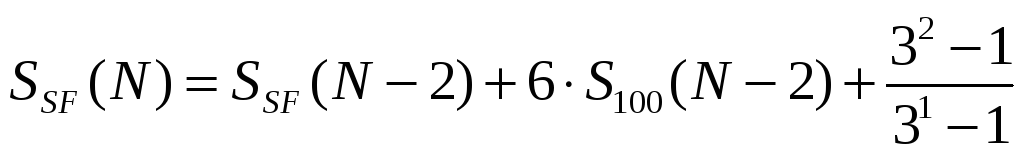. (14)
Equation 14 is recursive (the N-th value can be evaluated if the N-2 value is known). So it takes some effort to come up with closed-form expressions.

The closed-form expression for the number of moves of the k-th disk when executing the SemiFree Algorithm is given by Equation 15: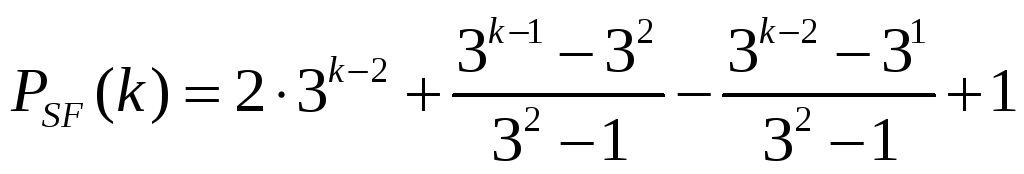; k odd

(15)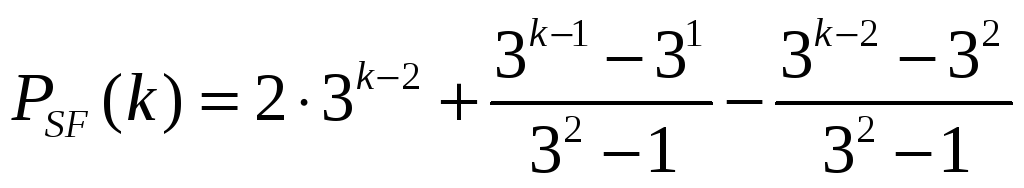; k even

The closed form expression for the total number of moves required to relocate a stack of N disks, executing the SemiFree Algorithm, is given by Equation 16: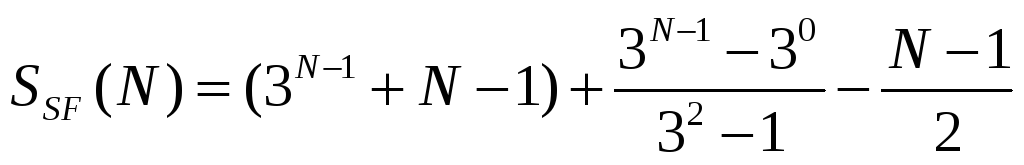; N odd

(16)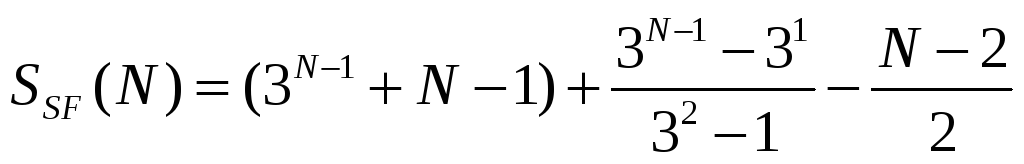; N even

Table 8 lists the number of moves of the k-th disk for the first eight stack "heights".

As shown, the number of SemiFree moves is generally larger than the equivalent "67" number of moves (refer to Table 6) but is generally significantly smaller than the equivalent "100" number of moves (refer to Table 4).

 k N 1 2 3 4 5 6 7 8 SUM 1 1 1 2 1 3 4 3 1 3 7 11 4 1 3 7 21 32 5 1 3 7 21 61 93 6 1 3 7 21 61 183 276 7 1 3 7 21 61 183 547 823 8 1 3 7 21 61 183 547 1641 2464